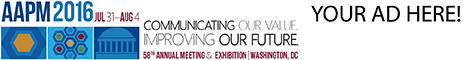# Program Information

## Impact of Approximating the Differential Photon Fluence On the Accuracy of the Spectroscopic Dose-Rate ConstantM Malin

## Presentations

#### TH-AB-BRA-8 (Thursday, July 16, 2015) 7:30 AM - 9:30 AM Room: Ballroom A

Purpose: To investigate why dose-rate constants computed using the spectroscopic technique do not agree with those computed using standard Monte Carlo (MC) techniques. Published work shows that the disagreement between the two techniques is not attributable to any currently characterized source of uncertainty. This work examined if approximations to the differential photon fluence made by the spectroscopic technique are the cause of the disagreement. Specifically, approximations of the spatial, angular and energy distributions of photons leaving the source were studied.

Methods: Phase spaces of the fluence leaving the encapsulation of 14 125-I and 6 103-Pd models were modified to reflect one of the approximated distributions while the other distributions were left unaltered. The dose-rate constant was computed from the modified phase space using MC and compared to that computed using standard MC techniques. This process was repeated for each assumption with each source model.

Results: Approximations to the differential photon fluence used in the spectroscopic technique cause offsets in the dose-rate constant of up to 5.3% relative to standard MC techniques. The largest offsets were introduced by the approximated angular fluence distribution. Approximations of the spatial distribution leaving the encapsulation caused smaller discrepancies with an average discrepancy of -0.4% relative to standard MC techniques. Approximations to the energy distribution introduced an average discrepancy of 0.1% relative to standard MC techniques, which is within the uncertainty of the computations.

Conclusion: Current approximations of the differential photon fluence used in the spectroscopic technique reduce the accuracy of the technique and are a source of uncertainty not currently considered with the technique. Either more accurate fluence distributions need to be used when computing the dose-rate constant with the spectroscopic technique, or the additional uncertainties arising from the approximated distributions need to be included when using the current calculation technique.

Contact Email: